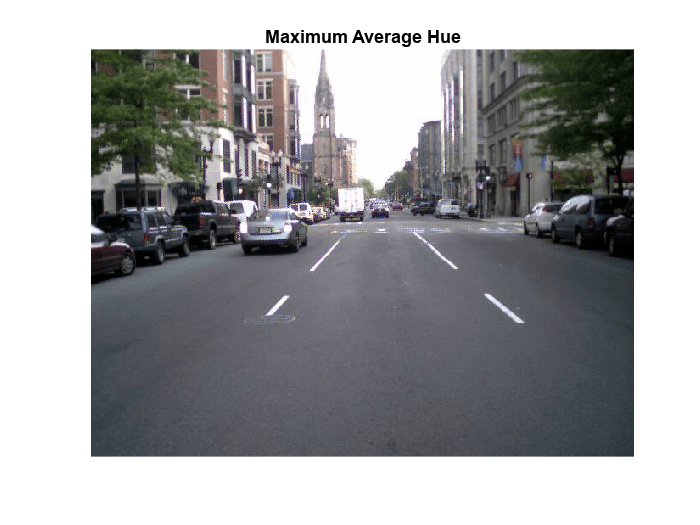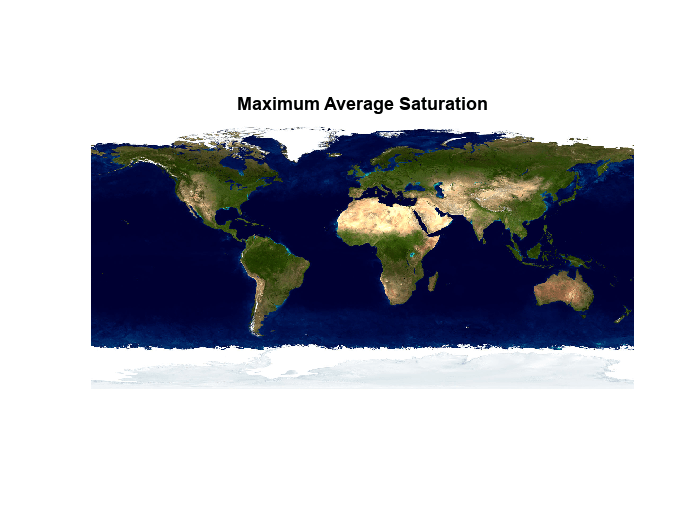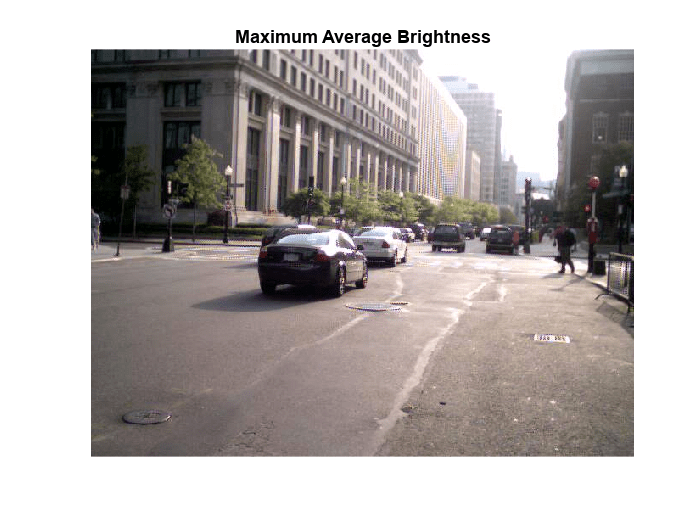# Compute Maximum Average HSV of Images with MapReduce

This example shows how to use `ImageDatastore` and `mapreduce` to find images with maximum hue, saturation and brightness values in an image collection.

### Prepare Data

Create a datastore using the images in `toolbox/matlab/demos` and `toolbox/matlab/imagesci`. The selected images have the extensions `.jpg`, `.tif` and `.png`.

```demoFolder = fullfile(matlabroot, 'toolbox', 'matlab', 'demos'); imsciFolder = fullfile(matlabroot, 'toolbox', 'matlab', 'imagesci');```

Create a datastore using the folder paths, and filter which images are included in the datastore using the `FileExtensions` Name-Value pair.

```ds = imageDatastore({demoFolder, imsciFolder}, ... 'FileExtensions', {'.jpg', '.tif', '.png'});```

### Find Average Maximum HSV from All Images

One way to find the maximum average hue, saturation, and brightness values in the collection of images is to use `readimage` within a for-loop, processing the images one at a time. For an example of this method, see Read and Analyze Image Files.

This example uses `mapreduce` to accomplish the same task, however, the `mapreduce` method is highly scalable to larger collections of images. While the for-loop method is reasonable for small collections of images, it does not scale well to a large collection of images.

### Scale to MapReduce

• The `mapreduce` function requires a map function and a reduce function as inputs.

• The map function receives blocks of data and outputs intermediate results.

• The reduce function reads the intermediate results and produces a final result.

### Map Function

• In this example, the map function stores the image data and the average HSV values as intermediate values.

• The intermediate values are associated with 3 keys, `'Average Hue'`, `'Average Saturation'` and `'Average Brightness'`.

```function hueSaturationValueMapper(data, info, intermKVStore) if ~ismatrix(data) hsv = rgb2hsv(data); % Extract Hue values h = hsv(:,:,1); % Extract Saturation values s = hsv(:,:,2); % Extract Brightness values v = hsv(:,:,3); % Find average of HSV values avgH = mean(h(:)); avgS = mean(s(:)); avgV = mean(v(:)); % Add intermediate key-value pairs add(intermKVStore, 'Average Hue', struct('Filename', info.Filename, 'Avg', avgH)); add(intermKVStore, 'Average Saturation', struct('Filename', info.Filename, 'Avg', avgS)); add(intermKVStore, 'Average Brightness', struct('Filename', info.Filename, 'Avg', avgV)); end end ```

### Reduce Function

• The reduce function receives a list of the image file names along with the respective average HSV values and finds the overall maximum values of average hue, saturation and brightness values.

• `mapreduce` only calls this reduce function 3 times, since the map function only adds three unique keys.

• The reduce function uses `add` to add a final key-value pair to the output. For example, `'Maximum Average Hue'` is the key and the respective file name is the value.

```function hueSaturationValueReducer(key, intermValIter, outKVSTore) maxAvg = 0; maxImageFilename = ''; % Loop over values for each key while hasnext(intermValIter) value = getnext(intermValIter); % Compare values to determine maximum if value.Avg > maxAvg maxAvg = value.Avg; maxImageFilename = value.Filename; end end % Add final key-value pair add(outKVSTore, ['Maximum ' key], maxImageFilename); end ```

### Run MapReduce

Use `mapreduce` to apply the map and reduce functions to the datastore, `ds`.

`maxHSV = mapreduce(ds, @hueSaturationValueMapper, @hueSaturationValueReducer);`
```******************************** * MAPREDUCE PROGRESS * ******************************** Map 0% Reduce 0% Map 12% Reduce 0% Map 25% Reduce 0% Map 37% Reduce 0% Map 50% Reduce 0% Map 62% Reduce 0% Map 75% Reduce 0% Map 87% Reduce 0% Map 100% Reduce 0% Map 100% Reduce 33% Map 100% Reduce 67% Map 100% Reduce 100% ```

`mapreduce` returns a datastore, `maxHSV`, with files in the current folder.

Read and display the final result from the output datastore, `maxHSV`. Use `find` and `strcmp` to find the file index from the `Files` property.

```tbl = readall(maxHSV); for i = 1:height(tbl) figure; idx = find(strcmp(ds.Files, tbl.Value{i})); imshow(readimage(ds, idx), 'InitialMagnification', 'fit'); title(tbl.Key{i}); end```### Local Functions

Listed here are the map and reduce functions that `mapreduce` applies to the data.

```function hueSaturationValueMapper(data, info, intermKVStore) if ~ismatrix(data) hsv = rgb2hsv(data); % Extract Hue values h = hsv(:,:,1); % Extract Saturation values s = hsv(:,:,2); % Extract Brightness values v = hsv(:,:,3); % Find average of HSV values avgH = mean(h(:)); avgS = mean(s(:)); avgV = mean(v(:)); % Add intermediate key-value pairs add(intermKVStore, 'Average Hue', struct('Filename', info.Filename, 'Avg', avgH)); add(intermKVStore, 'Average Saturation', struct('Filename', info.Filename, 'Avg', avgS)); add(intermKVStore, 'Average Brightness', struct('Filename', info.Filename, 'Avg', avgV)); end end %------------------------------------------------------------------------------------------ function hueSaturationValueReducer(key, intermValIter, outKVSTore) maxAvg = 0; maxImageFilename = ''; % Loop over values for each key while hasnext(intermValIter) value = getnext(intermValIter); % Compare values to determine maximum if value.Avg > maxAvg maxAvg = value.Avg; maxImageFilename = value.Filename; end end % Add final key-value pair add(outKVSTore, ['Maximum ' key], maxImageFilename); end %------------------------------------------------------------------------------------------```